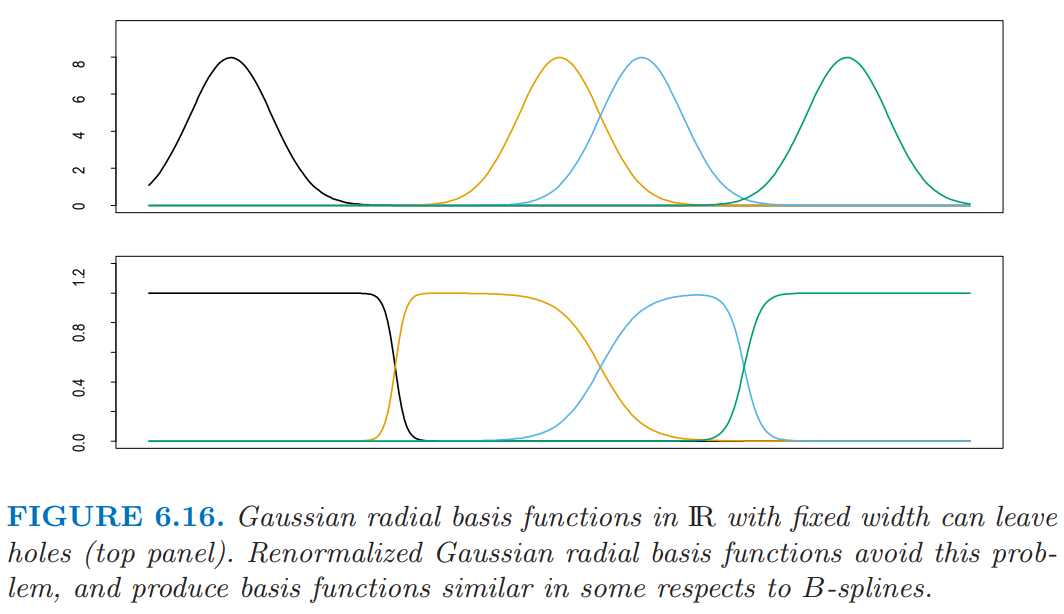6.7 径向基函数和核¶

• 优化关于所有参数的平方和

​ 这个模型一般称为 RBF 网络，这是 S 型神经网络的替代选择，将在第 11 章讨论；$\xi_j$ 和 $\lambda_j$ 在参数中有重要作用．这个准则是有着多重局部最小点的非凸函数，并且优化的算法类似神经网络中的算法．

• 分开估计 $\{\lambda_j,\xi_j\}$ 和 $\beta_j$．给定前者，后者的估计是简单的最小二乘问题．通常单独用 $X$ 的分布，以非监督的方式选择核参数 $\lambda_j$ 和 $\xi_j$．其中一种方式是在给定中心 $\xi_j$ 和缩放 $\lambda_j$ 时，对训练 $x_i$ 拟合高斯混合密度模型．更特别指定（adhoc）的方式使用聚类方法来确定原型 $\xi_j$，并且将 $\lambda_j=\lambda$ 看成是超参数．这些方式的显然缺点是条件分布 $\Pr(Y\mid X)$，并且特别地，$\E(Y\mid X)$ 在集中的地方是没有作用的．在正的一侧，可以更简单地实现．$\IR^p$ 中的 Nadaraya-Watson 核回归估计量 (6.2) 可以看成是重标准化后径向基函数的展开，

weiya 注：Recall